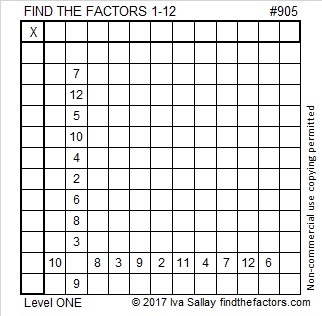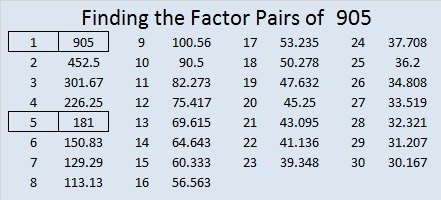# 905 and Level 1

905 is the sum of the seventeen prime numbers from 19 to 89.

905 is also the sum of these seven consecutive prime numbers:

• 109 + 113 + 127 + 131 + 137 + 139 + 149 = 905

Here’s today’s puzzle:Print the puzzles or type the solution on this excel file: 12 factors 905-913

29² + 8² = 905, and 28² + 11² = 905, making 905 the hypotenuse of four Pythagorean triples:

• 95-900-905, which is 5 times (19-180-181)
• 464-777-905, computed from 2(29)(8), 29² – 8², 29² + 8²
• 543-724-905, which is (3-4-5) times 181
• 616-663-905, computed from 2(28)(11), 28² – 11², 28² + 11²

The numbers in red are factors of 905.

• 905 is a composite number.
• Prime factorization: 905 = 5 × 181
• The exponents in the prime factorization are 1 and 1. Adding one to each and multiplying we get (1 + 1)(1 + 1) = 2 × 2 = 4. Therefore 905 has exactly 4 factors.
• Factors of 905: 1, 5, 181, 905
• Factor pairs: 905 = 1 × 905 or 5 × 181
• 905 has no square factors that allow its square root to be simplified. √905 ≈ 30.0832179This site uses Akismet to reduce spam. Learn how your comment data is processed.